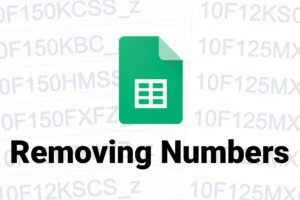# Why isn’t ISNUMBER working in sheets?

Recently, I tried to build a formula in sheets to tell me whether or not a character in a specific position of a string was a number or not. Turns out that none of them were? Even though, plain as day… there they are. Numbers.

You can see in fig. 1 that I’m using a formula to check and see if the character in the position all the way to the right is a number or not. However, I’m missing a crucial part in my formula:

``=ISNUMBER(RIGHT(D3,1))``

I’m forgetting to tell Sheets to see the numbers as numbers. I’m missing the VALUE command. According to Google Docs Support, the VALUE command converts a string in any of the date, time, or number formats that Google Sheets understands into a number. So, let’s give it a try using this formula, with value:

``=ISNUMBER(VALUE(RIGHT(D3,1)))``

As, you can see in fig. 2, that worked!

##### The Breakdown
• =ISNUMBER( = Checks whether a VALUE is a number.
• VALUE( = Converts a string into a format that Google Sheets understands as a number.
• RIGHT( = Tells Sheets to display all the characters from the right, the first VALUE “D3” is the CELL I was searching, and the second “1” is the number of spaces I want to display.

### Related posts

##### Removing HTML from a Cell in Google Sheets##### Counting in Google Sheets for SEO